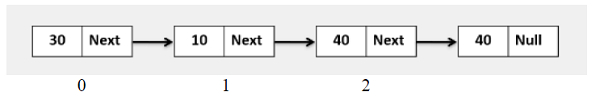# Golang program to update the ith index node value, when index is at the nth position, i.e., out of index bound.

Go ProgrammingServer Side ProgrammingProgramming

## ExampleApproach to solve this problem

Step 1 − Define a method that accepts the head of a linked list.

Step 3 − Initialize the index as i := 0.

Step 5 − If index i matches with the given index (to be updated), then update that node.

Step 6 − Else, return head.

## Example

Live Demo

package main
import "fmt"
type Node struct {
value int
next *Node
}
func NewNode(value int, next *Node) *Node{
var n Node
n.value = value
n.next = next
return &n
}
for temp != nil {
fmt.Printf("%d ", temp.value)
temp = temp.next
}
fmt.Println()
}
func UpdateKthIndexNode(head *Node, index , data int) *Node{
}
i := 0
for temp != nil{
if i == index{
temp.value = data
break
}
i++
temp = temp.next
}
}
func main(){
head := NewNode(30, NewNode(10, NewNode(40, NewNode(40, nil))))
}
Input Linked list is: 30 10 40 40
Update 10th index node, Linked List is: 30 10 40 40